# Problem 52844. Easy Sequences 42: Areas of Non-constructible Polygons

A constructible polygon is a regular polygon that can be constructed using only a compass and a straightedge.
Amazingly, Gauss found a way to identify which regular n-gon (abbreviation for a polygon, with n being the number of sides) is constructible, without even attempting to construct the polygon. Gauss's theorem states that an n-gon is contractible if and only if the totient of n is a power of 2. (The Euler Totient Function of a number n is defined as the number of integers from 1 to n that are coprime to n.)
For example, the 3-gon (equilateral triangle) is constructible because the totient of 3 is 2. Similarly, the 5-gon (regular pentagon) is constructible because the totient of 5 is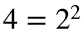. While, the 21-gon is non-constructible since the totient of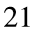is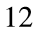, not a power of 2.
For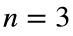to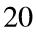, the number of sides of the n-gons that are constructible are as follows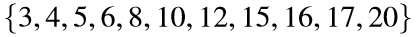and their totients,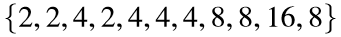, are all powers of 2. The non-constructible n-gons from 3 toare: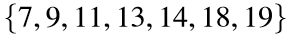, and their totients are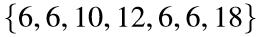.
Given the limit of the number of sides m, write a function that will output the sum of the areas of all non-constructible regular n-gons, for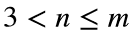, inscribed in a unit circle (i.e.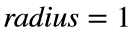).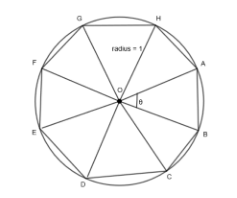NOTES:
• Equality in float class is hard to establish. Therefore, for consistency, please round-off each area to 4 decimal places, before taking the total.
• For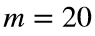, the function should return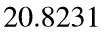, because the sum of areas of regular polygons with sides=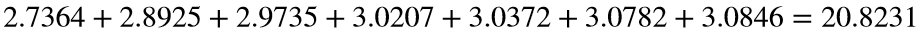.

### Solution Stats

80.0% Correct | 20.0% Incorrect
Last Solution submitted on Aug 04, 2023

### Community Treasure Hunt

Find the treasures in MATLAB Central and discover how the community can help you!

Start Hunting!